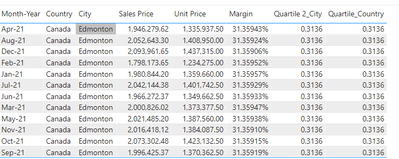cancel
Showing results for
Did you mean:

Fabric is Generally Available. Browse Fabric Presentations. Work towards your Fabric certification with the Cloud Skills Challenge.Helper I

## DAX - Percentile Calculation at different granularity

Hello,

I have a data set which is at multiple granularity - Region, Country, City, Daily with Profit as my measure

Now I want to calculate percentiles at different grain dynamically.My Dax is as follows -

CALCULATE(PERCENTILEX.EXC(VALUES(Sheet1[City]),[Margin],.5))

CALCULATE(PERCENTILEX.EXC(VALUES(Sheet1[Country]),[Margin],.5))

For example - 1) top 50 percentile for each city for each month

2) top 50 percentile for each country in a region for each month

I have been able to get this to work for each country and city for all months but the percentiles need to be applied on a monthly basis.

Thank you

13 REPLIES 13Community Support

Hi @2366

Do you mean you want to get Percentile_City and Percentile_Country for each month?If yes, try to filter the table like bellow,

``````CALCULATE(PERCENTILEX.EXC(VALUES(Sheet1[City]),[Margin],.5),filter(All(Sheet1),Sheet1[Month]=min(Sheet1[Month]) && Sheet1[City]=min(Sheet1[City])))
CALCULATE(PERCENTILEX.EXC(VALUES(Sheet1[Country]),[Margin],.5),filter(All(Sheet1),Sheet1[Month]=min(Sheet1[Month]) && Sheet1[Country]=min(Sheet1[Country])))``````

If this doesn't work, could you provide a sample file? Thanks

Best Regards,

Community Support Team _Tang

If this post helps, please consider Accept it as the solution to help the other members find it more quickly.MVP

Hi @2366

Try this:

CALCULATE(PERCENTILEX.EXC(ALLEXCEPT(Sheet1,Sheet1[City],Sheet1[Month]),[Margin],.5))

If this post helps, please consider accepting it as the solution to help the other members find it more quickly.Helper I

Hi @VahidDM ,

Thanks but it returns blank as it doesnt seem to calculate percentiles at Monthly/City grainMVP

@2366
Try this:

for City:
PERCENTILEX.EXC(Sheet1,[Margin],.5))
For Country :
CALCULATE(PERCENTILEX.EXC(Sheet1,[Margin],.5),removefilters(Sheet1[City]))

If this post helps, please consider accepting it as the solution to help the other members find it more quickly.Helper I

@VahidDM that worked partially but it appears the filter for month is also being removed.

I need the percentiles to be reset for every month/yearMVP

Do you have any Date table? what is the source of that Month Column?Helper I

No, I do not have an explicit Date table. I am using the inbuilt date hirearchy "Month" column in the visualMVP

So It would be better to add a Date table to your model then it will be easier to find a solution.
How to create a Date table:https://www.vahiddm.com/post/creating-calendar-table-with-3-steps

Can you share a sample of your PBIX file after removing sensetive data?Helper I

I have shared it in DM. I have applied the date table nowMVP

Try these two:

``````Quartile 2_City =
VAR _A =
SUMMARIZE( Sheet1, Sheet1[Date], Sheet1[City], "M", [Margin] )
RETURN
PERCENTILEX.EXC( _A, [M], .5 )``````

``````Quartile_Country =
VAR _A =
SUMMARIZE( Sheet1, Sheet1[Date], Sheet1[Country], "M", [Margin] )
RETURN
PERCENTILEX.EXC( _A, [M], .5 )``````

Output:If this post helps, please consider accepting it as the solution to help the other members find it more quickly.Helper I

Both of them are returning same values 😞MVP

Change Country to this:

``````Quartile_Country =
VAR _A =
calculatetable(SUMMARIZE( Sheet1, Sheet1[Date], Sheet1[Country], "M",[Margin]),removefilters(Sheet1[City]))
RETURN
PERCENTILEX.EXC( _A, [M], .5 )``````

If this post helps, please consider accepting it as the solution to help the other members find it more quickly.Helper I

Sorry, that doesn't seem to change eitherAnnouncements#### Power BI Monthly Update - November 2023

Check out the November 2023 Power BI update to learn about new features.#### The largest Power BI and Fabric virtual conference

130+ sessions, 130+ speakers, Product managers, MVPs, and experts. All about Power BI and Fabric. Attend online or watch the recordings.Top Solution Authors
Top Kudoed Authors
Users online (6,232)